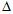# Electrical Engineering - Three-Phase Systems in Power Applications - Discussion

### Discussion :: Three-Phase Systems in Power Applications - General Questions (Q.No.5)

5.

In a-connected source feeding a Y-connected load,

 [A]. each phase voltage equals the difference of the corresponding load voltages [B]. each phase voltage equals the corresponding load voltage [C]. each phase voltage is one-third the corresponding load voltage [D]. each phase voltage is 60° out of phase with the corresponding load voltage

Explanation:

No answer description available for this question.

 Saminathan said: (Jun 18, 2011) In star to delta connection ph vt is equal to difference of line vt.

 Manimaran said: (Jul 28, 2011) Delta connection to starconection are equal to line voltage. vph=vl/3.

 Bitan said: (Aug 4, 2011) In delta connected source feeding a star connected load, phase voltage is equal to line voltage.

 Anusha said: (Apr 1, 2012) Whether the phase voltage of delta connected source equals the difference of line voltages of star connected load.

 Monu said: (Aug 3, 2012) Whether the phase voltage of delta connected source equals the difference of line voltages of star connected load. How?

 Abhaya said: (Feb 15, 2013) Phase voltage of delta connected = line voltage of delta. Difference of voltage in star connected load = delta connected source voltage.

 Abhishek said: (Jan 17, 2014) Whenever the delta connected source is feeding star connected load the phase voltage is always equal to the line voltage of star connected load it means vdelta = vload of star connected.

 Himanshu said: (Jan 30, 2014) In delta connection phase voltage is equal to line voltage.

 Rahul said: (Apr 7, 2014) I couldn't understand. What is the meaning of difference in load voltage?

 Bernard said: (May 31, 2014) What is the meaning of diff in load voltage?

 San said: (Jun 30, 2014) In Y connection line voltage is 1.732 times of phase voltage.

 Vijay said: (Jul 13, 2014) Vab=Va-Vb IN STAR so phase of delta Vsorce=|Vab| bc & ca. But line current is simply kcl across the node.

 Jhantu Kumar Maji said: (Nov 19, 2014) Delta connection Line volume = Phase volume and Star connection Line volume = 1/3 Phase volume.

 Saravanan said: (Nov 28, 2014) Delta not equal to star.

 Subhendu said: (Aug 1, 2015) If the delta connected source is fed to the star connected load then phase difference is 120'. So that difference will be same.

 Khushal said: (Jan 18, 2016) Load voltage e.g. phase voltage on load side which is connected in star and source is delta. So line and phase volt is equal for this. Now, V(source line) = Vr-Vy (load phase) depends on nomination R, Y, B.

 Muhammad Aftab Rafique said: (Nov 7, 2016) Tthe difference of the corresponding load voltage means: Vab = Va - Vb. So phase voltage is Vab. The difference of load voltages is Va - Vb.

 Yash said: (Dec 27, 2018) It's C, not A because in delta there is 3 phase R, Y, & B.